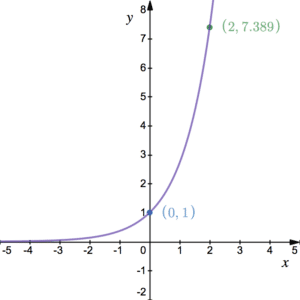# Differentiating exponentials and logarithmic functions

## Differentiating Exponentials

Differentiating exponentials usually requires the use of the chain rule. In the case of e to the x differentiating does not change the function, i.e. y and dy/dx are the same expression. The graph below shows the graph of $y=e^{x}$ where $e$, sometimes known as Euler’s number, is given by $e=2.718281828459$… See more on this type of graph. The number $e$ is special because everywhere on this graph, the gradient is the same as the $y$-coordinate.The derivative of $y=e^{kx}$, where $k$ is a constant, is $\frac{dy}{dx}=ke^{kx}$, i.e.:

$y=e^{kx},\hspace{10pt}\frac{dy}{dx}=ke^{kx}$

For $k=1$, this says that at each point on the graph of $y$, the gradient matches that of the $y$ coordinate:

$y=e^{x},\hspace{10pt}\frac{dy}{dx}=e^{x}$

Be careful when differentiating multiples of these functions. The power and multiple must be multiplied together. See the Examples. The StudyWell Differentiation eGuide has lots more on differentiation including exam style questions. To see how we find these derivatives, follow the process for differentiating $a^{kx}$ below.

## Differentiating $a^{kx}$

At higher level in mathematics, you may need to differentiate more complicated exponential functions. Suppose $y=a^{kx}$, where $a$ and $k$ are constants, the derivative is given by

$\frac{d}{dx}\left(a^{kx}\right)=ka^{kx}\ln(a)$

We can show this by letting $y=a^{kx}$, logging both sides first to get $\ln(y)=\ln\left(a^{kx}\right)=kx\ln(a)$ using a log rule then differentiating implicitly: $\frac{1}{y}\frac{dy}{dx}= k\ln(a)$ since $k\ln(a)$ is a constant. It follows that $\frac{dy}{dx}=ky\ln(a)=ka^{kx}\ln(a)$. See Example 4. This applies for all positive $a$ and all $k$. If we choose $a=e$ the exponential constant, then this tells us that $\frac{d}{dx}\left(e^{kx}\right)ke^{kx}$. Furthermore, if $k=1$ then we have $\frac{d}{dx}\left(e^x\right)=e^x$.

## Differentiating $\ln(x)$

As higher levels, you should know that the derivative of $\ln(x)$ is:

$\frac{d}{dx}\left(\ln(x)\right)=\frac{1}{x}$

We can show this using differentiation from first principles and the log rules:

$\frac{d}{dx}\left(\ln(x)\right)=\lim_{h\rightarrow 0}\frac{\ln(x+h)-\ln(x)}{h}=\lim_{h\rightarrow 0}\frac{\ln\left(\frac{x+h}{x}\right)}{h}=\lim_{h\rightarrow 0}\frac{1}{h}\ln\left(1+\frac{h}{x}\right)=\lim_{h\rightarrow 0}\ln\left(\left(1+\frac{h}{x}\right)^{\frac{1}{h}}\right)$

At this point we make a change of variables. Let $h=nx$ so that $h\rightarrow 0$ as $n\rightarrow 0$. It follows that

$\frac{d}{dx}\left(\ln(x)\right)=\lim_{n\rightarrow 0}\ln\left(\left(1+n\right)^{\frac{1}{nx}}\right)=\lim_{n\rightarrow 0}\frac{1}{x}\ln\left(\left(1+n\right)^{\frac{1}{n}}\right)=\frac{1}{x}\ln\left(\lim_{n\rightarrow 0}\left(\left(1+n\right)^{\frac{1}{n}}\right)\right)=\frac{1}{x}\times 1$

Note that we commute the multiplication by a factor of $\frac{1}{x}$ and logging with the limit (see more on limits). Also note that $\frac{d}{dx}\left(\ln(kx)\right)=\frac{1}{x}$ too, where $k$ is a constant. This is because we can write $\ln(kx)$ as $\ln(k)+\ln(x)$ using the log rules. Since $\ln(k)$ is also a constant for constant $k$ it differentiates to 0. See example 5.

## Examples of Differentiating Exponentials & Log functions

Differentiate $y=2e^{4x}$.

Given that $h(x)=\frac{1+20e^{5x}}{4e^{2x}}$,
find $h'(x)$.

A scientist drops a heated metal ball into a cooler liquid. After time $t$ seconds, the temperature, $T^\circ$, of the ball is

$T(t)=350e^{-0.1t}+27$.

Find the temperature of the ball at the instant the scientist drops the ball into the liquid. Find the rate at which the ball is cooling after 10 seconds.

Find the exact value of the gradient of the curve of $y=1-3^{2x}$ at the point where $x=\log_3(2)$.

Find the equation of the tangent to the curve of $f(x)=x^2-\frac{1}{2}\ln(x)$ at the point with $x$-coordinate 1. Give your answer in the form $ax+by+c=0$ where $a$, $b$ and $c$ are integers.